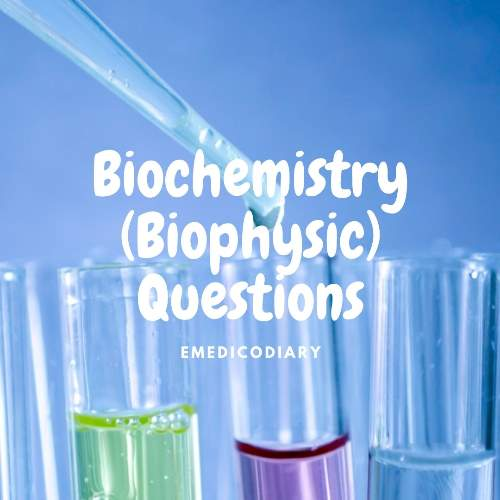### MBBS 1st Year Question Collection Of Biophysics (Biochemistry)

Biophysics

Short questions

1. Define PH?
2. Write down the properties of the pH scale?
3. Define pOH, What do you mean by Kw?
4. Define buffer. What is the blood buffer? What are the factors affecting buffering capacity? Which one is a prime buffer and why?
5. Define buffer system . Why bicarbonate buffer is the most important buffer in the body?
6. Define standard solutions?
7. What are the ways to express the cons? Of solutions?
8. Define colloid solutions ? Give their properties and how colloid can be separate from the solution?
9. Define colloid and crystalloid with examples
10. What is membrane transport? Classify it
11. Define osmosis
12. Define titration. Role of indicator in titration.
13. Define the anion gap?
14. Define protective colloid?
15. The plasma phosphate buffer system is stronger than the plasma buffer system explains.
16. How Hb buffer act?
17. Define diffusion.
18. Enumerate buffer system present inside the red blood cell?
19. Define acid, base? Justify the pH of water is 7?
20. Define pK. How normal pH is maintained in the body?
21. Difference between colloidal and cristaloidals solutions?
22. What is tonicity
23. Why HCOC3 buffer is the prime buffer and PO4 buffer is a stronger buffer?

1. State and explain with importance. Law of mass action.
2. State and explain with importance. Henderson Hassle Balch equation.
3. State and explain with important Gibbs Donan membrane equilibrium.
4. Define and classify solutions. Mention of expressing solutions concentration.
5. A) Active and passive transport. B) Simple and facilitated diffusion.strong and week acid. D) Colloid from crystalloid.
6. Define and classify Active transport.
7. Define isotope with important. Give the difference between isotope and isomers.
8. Define SI units. Write the basic unit with a symbol.
9. What are the serial dilutions?
10. Define biological membranes. What are the processes of transport through biological membranes with examples?
11. Short note: isotope
12. Define isomers with examples

Calculation

1. Calculate how much NaCl required preparing 500ml 500 normal solutions and 1 little normal saline.
2. How much H2804 is required for 100ml of N/10 H2804 solutions?
3. Give the calculation to prepare 100ml of N/10 H2804 in the laboratory.
4. How will you prepare 200ml of isotonic solutions? Short note 1) normal solutions.2surface tension 3 viscosity 4 colligative properties of solutions.5 tonicity 6 dialysis 7 isotopes.
5. Osmolarity and molarity of 9ml solutions of NaCl.